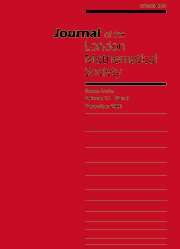Skip to main content Accessibility help
Home
Hostname: page-component-684bc48f8b-l9xz9 Total loading time: 0.295 Render date: 2021-04-14T01:47:49.359Z Has data issue: true Feature Flags: { "shouldUseShareProductTool": true, "shouldUseHypothesis": true, "isUnsiloEnabled": true, "metricsAbstractViews": false, "figures": false, "newCiteModal": false, "newCitedByModal": true }Journal of the London Mathematical Society

# HERMAN RINGS AND ARNOLD DISKS

Published online by Cambridge University Press:  08 December 2005

## Abstract

For $(\l,a)\in \matbb{C}^*\times \mathbb{C}$, let $f_{\lambda,a}$ be the rational map defined by $f_{\lambda,a}(z) \,{=}\, \lambda z^2 {(az+1)/(z+a)}$. If $\alpha\in \mathbb{R}/\mathbb{Z}$ is a Brjuno number, we let ${\cal D}_\alpha$ be the set of parameters $(\lambda,a)$ such that $f_{\lambda,a}$ has a fixed Herman ring with rotation number $\alpha$ (we consider that $({\it e}^{2i\pi\alpha}{,}0)\,{\in}\, {\cal D}_\alpha$). Results obtained by McMullen and Sullivan imply that, for any $g\in {\cal D}_\alpha$, the connected component of ${\cal D}_\alpha\cap (\mathbb{C}^*\times(\mathbb{C}\setminus \{0,1\}))$ that contains g is isomorphic to a punctured disk.

We show that there is a holomorphic injection $\cal{F}_\alpha\,{:}\,\mathbb{D}\,{\longrightarrow}\, {\cal D}_\alpha$ such that $\cal{F}_\alpha(0) = ({\it e}^{2i\pi \alpha},0)$ and $\cal{F}_\alpha'(0)=(0,r_\alpha),$ where $r_\alpha$ is the conformal radius at 0 of the Siegel disk of the quadratic polynomial $z\longmapsto {\it e}^{2i\pi \alpha}z(1+z)$.

As a consequence, we show that for $a\in (0,1/3)$, if $f_{\l,a}$ has a fixed Herman ring with rotation number $\alpha$ and if $m_a$ is the modulus of the Herman ring, then, as $a\,{\rightarrow}\,0$, we have ${\it e}^{\pi m_a} \,{=} ({r_\alpha}/{a}) + {\cal O}(a).$

We finally explain how to adapt the results to the complex standard family $z\,{\longmapsto} \lambda z {\it e}^{({a}/{2})(z-1/z)}$.

## Keywords

Type
Notes and Papers
Information
Journal of the London Mathematical Society , December 2005 , pp. 689 - 716
Copyright
The London Mathematical Society 2005

## Access options

Get access to the full version of this content by using one of the access options below.

### Full text views

Full text views reflects PDF downloads, PDFs sent to Google Drive, Dropbox and Kindle and HTML full text views.

Total number of HTML views: 0
Total number of PDF views: 6 *
View data table for this chart

* Views captured on Cambridge Core between September 2016 - 14th April 2021. This data will be updated every 24 hours.

# Send article to Kindle

To send this article to your Kindle, first ensure no-reply@cambridge.org is added to your Approved Personal Document E-mail List under your Personal Document Settings on the Manage Your Content and Devices page of your Amazon account. Then enter the ‘name’ part of your Kindle email address below. Find out more about sending to your Kindle. Find out more about sending to your Kindle.

Note you can select to send to either the @free.kindle.com or @kindle.com variations. ‘@free.kindle.com’ emails are free but can only be sent to your device when it is connected to wi-fi. ‘@kindle.com’ emails can be delivered even when you are not connected to wi-fi, but note that service fees apply.

Find out more about the Kindle Personal Document Service.

HERMAN RINGS AND ARNOLD DISKS
Available formats
×

# Send article to Dropbox

To send this article to your Dropbox account, please select one or more formats and confirm that you agree to abide by our usage policies. If this is the first time you use this feature, you will be asked to authorise Cambridge Core to connect with your <service> account. Find out more about sending content to Dropbox.

HERMAN RINGS AND ARNOLD DISKS
Available formats
×

# Send article to Google Drive

To send this article to your Google Drive account, please select one or more formats and confirm that you agree to abide by our usage policies. If this is the first time you use this feature, you will be asked to authorise Cambridge Core to connect with your <service> account. Find out more about sending content to Google Drive.

HERMAN RINGS AND ARNOLD DISKS
Available formats
×
×

#### Conflicting interests

Do you have any conflicting interests? *